# Accelerate Motion Planning with Deep-Learning-Based Sampler

The example demonstrates how to augment sampling-based planners such as RRT (rapidly-exploring random tree) and RRT* with a deep-learning-based sampler to find optimal paths efficiently.

The classical sampling-based planners such as RRT and RRT* rely on generating samples from a uniform distribution over a specified state space. However, these planners typically restrict the actual robot path to a small portion of the state space. The uniform sampling causes the planner to explore many states which do not have an impact on the final path. This causes the planning process to become slow and inefficient, especially for state spaces with a large number of dimensions.

You can train a deep learning network to generate learned samples that can bias the path towards the optimal solution. This example implements the approach proposed by Ichter et al. in their paper titled "Learning Sampling Distributions for Robot Motion Planning". This approach implements a Conditional Variation Autoencoder (CVAE) that generates learned samples for a given map, start state, and goal state. The Train Deep Learning-Based Sampler for Motion Planning example explains the architecture of the deep learning network and the training pipeline.

RRT* Path (with Uniform Sampling):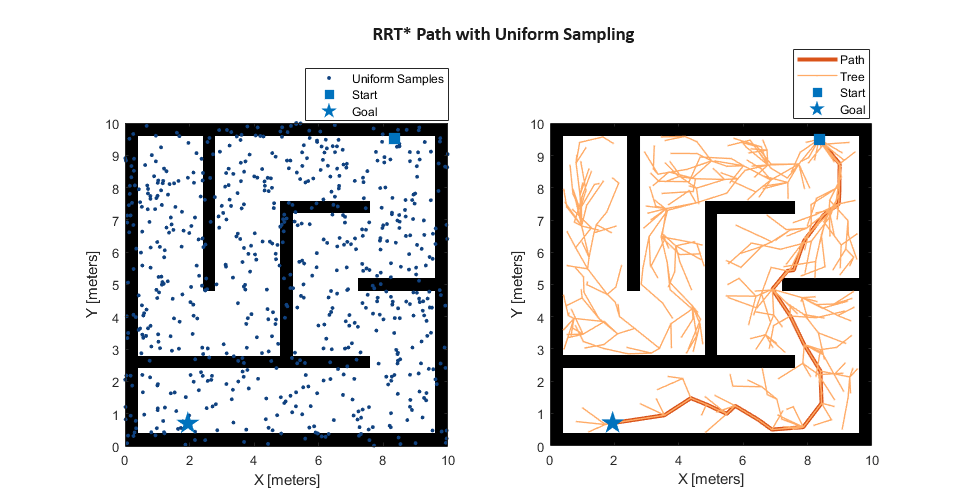RRT* Path (with Learned Sampling):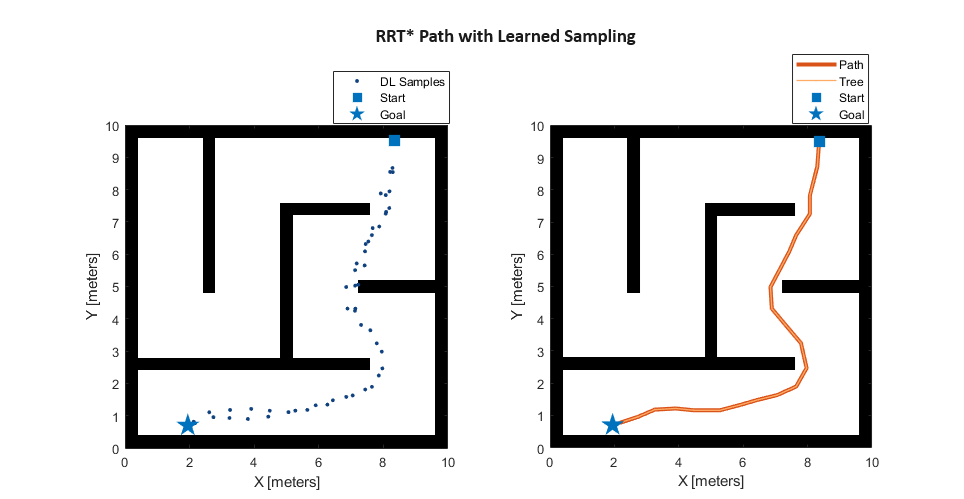Load the pretrained network from the mat file `CVAESamplerTrainedModel.mat`. The network was trained using the dataset `MazeMapDataset.mat`. The Train Deep Learning-Based Sampler for Motion Planning example explains the network training.

`load("CVAESamplerTrainedModel","decoderNet")`

Load the dataset from the mat file `MazeMapDataset.mat`. The dataset contains 2000 maze maps and their corresponding start states, goal states, and path states.

`load("MazeMapDataset","dataset","mapParams")`

#### Dataset Generation

The dataset was generated using the `examplerHelperGenerateData` function. The function uses the `mapMaze` function for the generation of maps and randomly samples start and goal states from a set of start and goal states. For more details, see the Dataset Generation section in the Train Deep Learning-Based Sampler for Motion Planning example.

#### Prediction Data

Select the part of the dataset that has been allocated for testing that corresponds to the last (`1-split`) fraction. As a result, the size of the test dataset is 200 out of 2000.

```split = 0.9; testInd = floor(split*length(dataset))+1:length(dataset); dataset = dataset(testInd);```

#### Visualize Maps

Visualize four random maps and their start and goal states from the test dataset.

```figure(Name="Maps"); for i=1:4 subplot(2,2,i) ind = randi(length(dataset)); [map,start,goal] = exampleHelperGetData(dataset,ind); exampleHelperPlotData(map,start,goal); end```### Create Custom State Space with Deep-Learning-Based Sampler

The `ExampleHelperCustomStateSpaceSE2` class defines the custom SE(2) state space for the learned sampling. The class inherits `stateSpaceSE2` and overloads the `sampleUniform` function to generate a combination of learned and uniform samples based on the value of the `Lambda` property. CVAE Decoder network generates learned samples in the `sampleUniform` function, while the `sampleUniform` function of `stateSpaceSE2` generates uniform samples.

This class constructor takes the inputs `start`, `goal`, `map`, `maxSamples`, and `network`. Using these constructor inputs pregenerate `maxSamples`, the number of learned samples at constructor call, to reduce the number of CVAE Decoder network calls and speed-up `plan` function of `plannerRRTStar` object. Because the `plan` function generates one-one sample for each iteration of it for custom state space. As a result, to avoid the CVAE Decoder network in the `plan` function, set `maxSamples` to the `MaxIterations` property of `plannerRRTStar` object. Plan an optimal path with few samples using the custom state space and `validatorOccupancyMap` object.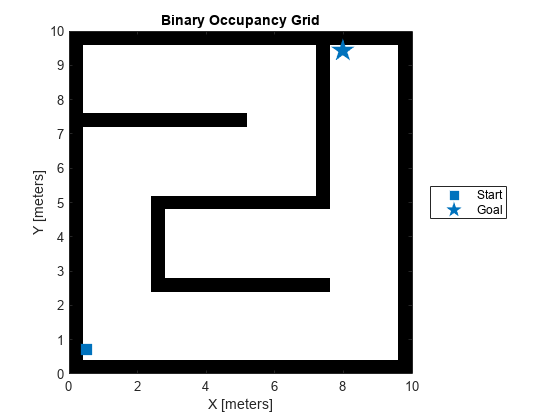Obtain the `start`, `goal`, and `map` data from the dataset already loaded in the Load Dataset section.

The `network` contains the CVAE Decoder network already loaded in the Load Pretrained Network section. Generate samples corresponding to `maxSamples` using this network. For more details about the CVAE network, see Define Network Architecture section of Train Deep Learning-Based Sampler for Motion Planning example. The decoder takes the inputs `start`, `goal`, `map` and latent state $z$ sampled from the normal distribution $N\left(0,I\right)$.

The `network` also takes the autoencoder network that encodes the maze type of maps into a compact representation, to speed up the training in the Train Deep Learning-Based Sampler for Motion Planning example.

Load the pretrained autoencoder model from the mat file `MapsAutoencoder.mat`.

```% Load autoencoder network that encodes maze maps load("MazeMapAutoencoder.mat","mapsAE") % Prepare network network = struct("DecoderNet",decoderNet,"MapsAutoEncoder",mapsAE);```

### Run RRT* with Custom State Space

Run the `plannerRRTStar` with `ExampleHelperCustomStateSpaceSE2` for the test dataset loaded in the Load Dataset section. We can vary the `testInd` value to switch different maps in the test data. You can vary the `lambda` value in range [0, 1] to observe the effect of learned sampling proportion on the final results.

You can confirm from the following results that the learning sampling helps the `plannerRRTStar` to efficiently find optimal paths between the start and the goal.

• For the lower `lambda` value, the RRT tree is spread across a larger region of the map and the path is less optimal.

• For the higher `lambda` value, the RRT tree is concentrated towards the learned samples and the path is more optimal. Also, lambda value less than one guarantees completeness.

Initialize state-space, validator, and planner input parameters.

```% Select test data index (1-200) testInd =54; % Get map, start, goal for current test index [map,start,goal] = exampleHelperGetData(dataset,testInd); % Select the learned sampling proportion 0 = pure uniform, 1 = pure learned lambda =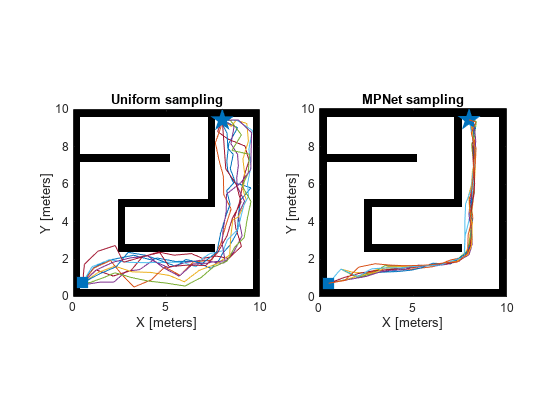0.7; %Set max Iterations maxIters = 1000;```

Create the custom state space and state validator objects. Integrate these objects to `plannerRRTStar` object and plan a path with the `plan` object function.

```% Set random seed rng("default"); % Create ExampleHelperCustomStateSpaceSE2 customSE2 = ExampleHelperCustomStateSpaceSE2(start,goal,map,maxIters,network); customSE2.StateBounds = [map.XWorldLimits;map.YWorldLimits;[-pi,pi]]; customSE2.Lambda = lambda; % Create stateValidator sv = validatorOccupancyMap(customSE2); sv.Map = map; sv.ValidationDistance = 0.1; % Create plannerRRTStar planner = plannerRRTStar(customSE2,sv); planner.MaxConnectionDistance = 1; planner.MaxIterations = maxIters; % Run the planner [pathObj,solnInfo] = plan(planner,start,goal); % Visualize the results figure(Name="RRT results") exampleHelperPlotData(map,start,goal,pathObj,solnInfo);```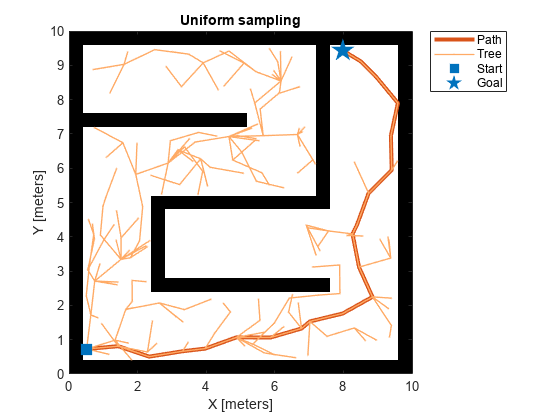### Evaluation Metrics

Analyze the evaluation metrics such as Success Rate and Path Costs by running the `plannerRRTStar` with `ExampleHelperCustomStateSpaceSE2` which contains the deep-learning-based sampler. To obtain robust metrics, perform 100 runs for each chosen map, start and goal combination.

Compare the evaluation metrics between learned sampling (with `λ=0.5`) and uniform sampling. Note that for the comparison, use `ExampleHelperCustomStateSpaceSE2` for learned sampling and `stateSpaceSE2` for uniform sampling. The `Lambda` property of the `ExampleHelperCustomStateSpaceSE2` class represents the proportion of learning-based samples (`λ`).

• The default `Lambda` value is `0.5` which means the probability of learned and uniform sampling is equal.

• If `Lambda` is `0`, the `sampleUniform` function will sample states uniformly.

• If `Lambda` is `1`, the `sampleUniform` function will sample only from learned samples.

The planner results vary depending on the value of the `Lambda` property. You can set the `Lambda` property as shown in the following code snippet.

```% Set Lambda = 0.9 (~50% of samples are learned samples) customSE2.Lambda = 0.5 ```

Choose few maps from the test data that have two or more turns from left to right or vice-versa. For these maps, `plannerRRTStar` with uniform sampling frequently fails to find the path if the number of samples are less than or equal to 500. The `examplerHelperPickTestDataForEvaluation` function loads the selected maps and the corresponding start and goal states.

`[maps,startStates,goalStates] = examplerHelperPickTestDataForEvaluation(dataset);`

Visualize data used for extracting evaluation metrics.

```figure(Name="Maps For Evaluation Metrics"); for i=1:5 subplot(2,3,i) exampleHelperPlotData(maps{i},startStates(i,:),goalStates(i,:)) end```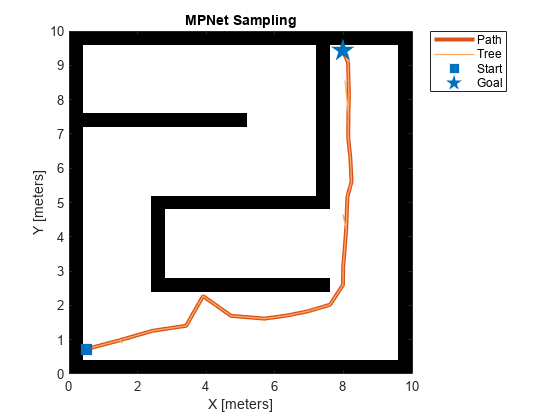#### Success Rate

Define the success rate as the fraction of total runs for which the paths are found. Compare the success rate between learned sampling (with `λ=0.5`) and uniform sampling (with `λ=0`). You can observe that rise in success rate is sharp for learned sampling as compared to uniform sampling. The learned sampling achieves the success rate of 90% at around 400 samples, whereas uniform sampling only achieves the success rate of 90% at 1000 samples. This indicates that learned sampling helps achieve a higher success rate with a lesser number of samples.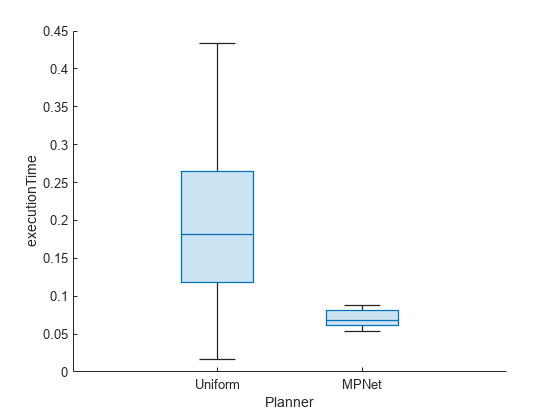Use the `exampleHelperSuccessRateEvaluation` function to extract the success rate metric. The following code snippet shows how to run this function to obtain the metric and the plots. Note that it took about 60 minutes to run this helper function on a Linux machine. Results may vary for your system.

```% Call success rate example helper function [successRateLearnedAvg,successRateUniformedAvg] = exampleHelperSuccessRateEvaluation(maps, ... startStates,goalStates,network); ```

In the `exampleHelperSuccessRateEvaluation` function, change the `seed` value for each run to get different results at each run, and compute the average success rate over 100 runs. Set `optimize` to `false`, so the `plannerRRTStar` stops after the path is found and does not optimize further. You can modify the maximum iterations, number of runs, lambda, etc. for evaluation.

```% Set optimize to false to exit from planner if path found. optimize = false; maxIterations = [5:50:500 1000:500:2500]; maxConnectionDistance = 1; lambda = 0.5; nRunTime = 100; seed = 100; % ... for i=1:numel(maxIterations) for j=1:nRunTime [successRateLearned(j,i),successRateUniformed(j,i)] = exampleHelperSuccessRateComputation(... maps,optimize,maxConnectionDistance,maxIterations(i),startStates,goalStates,network,lambda,seed+j*10); end end ```

#### Path Costs

Define path cost as the average cost for the total number of 100 runs for each maps choosen using `examplerHelperPickTestDataForEvaluation` function. Compare the success rate between learned sampling (with `λ=0.5`) and uniform sampling (with `λ=0`). You can observe that the rate of convergence of the path cost is very fast for the learned sampling as compared to the uniform sampling. For 500 samples, uniform sampling cannot find a path for each map and each run, while the learned sampling can, and the path is better than the uniform sampling path at 2500 samples.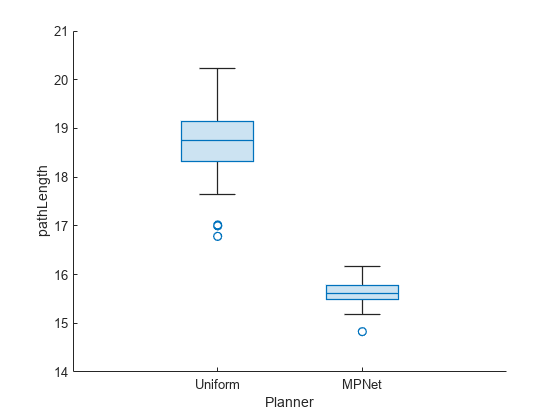Use the `exampleHelperPathCostEvaluation` function to extract the path cost. The following code snippet shows how to run this function to obtain the metrics and the plots. Note that it took about 6 hours to run this helper function on a Linux machine. Results may vary for your system.

```% Call execution time example helper function [pathCostsLearnedOptimized,pathCostsUniformedOptimized] = exampleHelperPathCostEvaluation(maps, ... startStates,goalStates,network) ```

In the `exampleHelperPathCostEvaluation` function, Set `optimize` to `true`, so that it continues to optimize for the fixed number of samples even if the goal is reached earlier. You can modify the maximum iterations, number of runs, lambda, etc. for evaluation.

```% Set optimize to false to exit from planner if path found. optimize = true; maxIterations = 500:500:2500; maxConnectionDistance = 1; lambda = 0.5; nRunTime = 2; seed = 100; %... for i=1:numel(maxIterations) for j=1:nRunTime [pathCostLrndOpt(j,i),pathCostUniOpt(j,i)] = exampleHelperPathCostComputation(maps,optimize, ... maxConnectionDistance,maxIterations(i),startStates,goalStates,network,lambda,seed+j*10); end end ```

### Conclusion

This example shows how to integrate the deep-learning-based sampler trained in the Train Deep Learning-Based Sampler for Motion Planning example with RRT* planner using a custom state space class. It shows how the planned path and the RRT tree, improve with the learned sampling. It also shows the learned sampling gives better performance using the evaluation metrics such as success rate and path cost.

### Bibliography

1. Ichter, Brian, James Harrison, and Marco Pavone. "Learning Sampling Distributions for Robot Motion Planning." In 2018 IEEE International Conference on Robotics and Automation (ICRA), 7087–94. Brisbane, QLD: IEEE, 2018. https://doi.org/10.1109/ICRA.2018.8460730.